# Volume of a Triangular Prism Online Quiz

#### Le Guide Définitif du Développement Web HTML.

76 Lectures 3.5 hours

#### SAP ERP : Gestion magasin WM (débutant et avancé)

66 Lectures 4.5 hours

#### Initiation SAP ERP débutant - Les Bases

12 Lectures 1 hours

Following quiz provides Multiple Choice Questions (MCQs) related to Volume of a Triangular Prism. You will have to read all the given answers and click over the correct answer. If you are not sure about the answer then you can check the answer using Show Answer button. You can use Next Quiz button to check new set of questions in the quiz.Q 1 - Find the volume of the following triangular prism.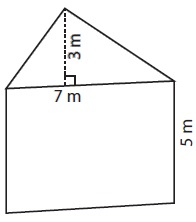### Explanation

Step 1:

Volume of a triangular prism = Areas of base triangle × height of prism

Step 2:

Volume of given prism V = $\frac{1}{2}$ × 7 × 3 × 5

= 52.5 cubic meter

Q 2 - Find the volume of the following triangular prism.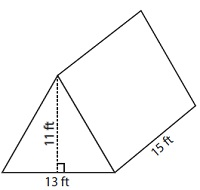### Explanation

Step 1:

Volume of a triangular prism = Areas of base triangle × height of prism

Step 2:

Volume of given prism V = $\frac{1}{2}$ × 13 × 11 × 15

= 1072.5 cubic feet

Q 3 - Find the volume of the following triangular prism.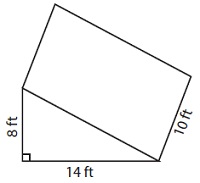### Explanation

Step 1:

Volume of a triangular prism = Areas of base triangle × height of prism

Step 2:

Volume of given prism V = $\frac{1}{2}$ × 14 × 8 × 10

= 560 cubic feet

Q 4 - Find the volume of the following triangular prism.### Explanation

Step 1:

Volume of a triangular prism = Areas of base triangle × height of prism

Step 2:

Volume of given prism V = $\frac{1}{2}$ × 6 × 4 × 12

= 144 cubic in

Q 5 - Find the volume of the following triangular prism.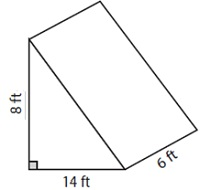### Explanation

Step 1:

Volume of a triangular prism = Areas of base triangle × height of prism

Step 2:

Volume of given prism V = $\frac{1}{2}$ × 14 × 8 × 6

= 336 cubic ft

Q 6 - Find the volume of the following triangular prism.### Explanation

Step 1:

Volume of a triangular prism = Areas of base triangle × height of prism

Step 2:

Volume of given prism V = $\frac{1}{2}$ × 3 × 8 × 3

= 36 cubic in

Q 7 - Find the volume of the following triangular prism.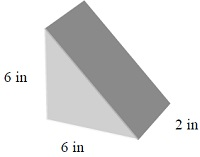### Explanation

Step 1:

Volume of a triangular prism = Areas of base triangle × height of prism

Step 2:

Volume of given prism V = $\frac{1}{2}$ × 6 × 6 × 2

= 36 cubic in

Q 8 - Find the volume of the following triangular prism.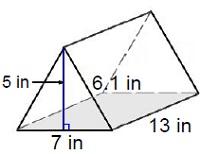### Explanation

Step 1:

Volume of a triangular prism = Areas of base triangle × height of prism

Step 2:

Volume of given prism V = $\frac{1}{2}$ × 7 × 5 × 13

= 227.5 cubic in

Q 9 - Find the volume of the following triangular prism.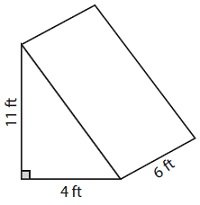### Explanation

Step 1:

Volume of a triangular prism = Areas of base triangle × height of prism

Step 2:

Volume of given prism V = $\frac{1}{2}$ × 4 × 11 × 6

= 132 cubic feet

Q 10 - Find the volume of the following triangular prism.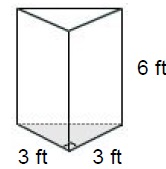### Explanation

Step 1:

Volume of a triangular prism = Areas of base triangle × height of prism

Step 2:

Volume of given prism V = $\frac{1}{2}$ × 3 × 3 × 6

= 27 cubic ft

volume_of_triangular_prism.htm